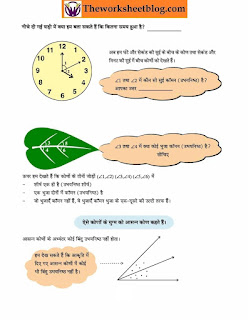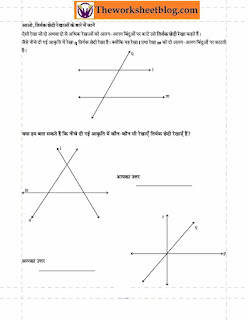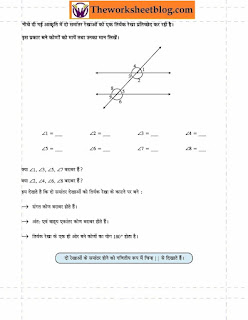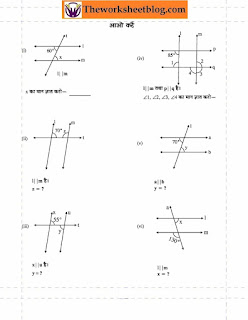Lines and Angles । NCERT 7th Math(रेखा एवं कोण)

The pairs of lines are nothing but the two lines, which may be intersects or parallel or perpendicular as shown below: Angles: The region between two infinitely long lines pointing a certain direction (ray) from a common point (or vertex) is termed as an angle.
The line has neither initial nor terminal points with indefinite length.The pairs of lines are nothing but the two lines, which may be intersects or parallel or perpendicular as shown below:Angles:

The region between two infinitely long lines pointing a certain direction (ray) from a common point (or vertex) is termed as an angle. That is, the amount of turn is measured by an angle, which can be commonly measured by an instrument called “protractor”. The symbol for an angle is “ ∠ ”.The angles are classified into several types based on the angle measures.1. Acute angle: Angle that is less than 90 °

2. Right angle: Angle that is exactly equal to 90 °

3. Obtuse angle: Angle that is greater than 90 ° and less than 180 °

4. Straight angle: Angle that is exactly equal to 180 °

5. reflex angle: Angle that is greater than 180 ° and less than 360 °

6. Complete (Full) angle: Angle that is exactly equal to 360 °

Lines and Angles Worksheet  for Hindi mediumLines and Angles  (रेखा एवं कोण) WorksheetLines and Angles  (रेखा एवं कोण) WorksheetLines and Angles  (रेखा एवं कोण) WorksheetLines and Angles  (रेखा एवं कोण) WorksheetLines and Angles  (रेखा एवं कोण) Worksheetworksheet on trianglesworksheet on trianglesproperties of triangles worksheetproperties of triangles worksheetlines and angles class 5 ,6,7,8 worksheetlines and angles class 5 ,6,7,8 worksheetlines and angles class 5 ,6,7,8 worksheetlines and angles free printable worksheets.lines and angles free printable worksheets.lines and angles free printable worksheets.
Lines and Angles:

If the lines or line segments intersects, then the angles are formed. The relations between angles are characterized by the pairs of angles as listed below:

1. Complementary angles - Sum of the two angles is right angle

2. Supplementary angles - - Sum of the two angles is straight angle

3. Vertical angles - Angles formed in the opposite direction

4. Linear pairs of angles - Alternate interior angles, alternate exterior angles and corresponding angles

Special angle pairs:

The angle between two parallel lines and a transversal lines form a special pairs of angles.Alternate interior angles: ∠ s , ∠ r , ∠ u , and ∠ t in which ∠ s = ∠ u and ∠ r= ∠ t

Alternate exterior angles: ∠ p , ∠ q , ∠ v , and &∠ w in which ∠ p = ∠ v and ∠ q= ∠ w

Corresponding angles: ∠ p = ∠ t , ∠ q = ∠ u , ∠ r = ∠ v , and ∠ s = ∠ w